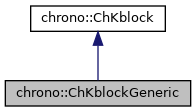chrono::ChKblockGeneric Class Reference

## Description

Class that represent nxn sparse blocks to put into K global matrix, that is blocks that connect N 'variables' and build a matrix K in a sparse variational inequality VI(Z*x-d,K):

Note that K blocks often have a physical interpretation as stiffness, but not always, for example they can represent hessians. Note that all blocks in K, all masses and constraint jacobians Cq are not really assembled in large matrices, so to exploit sparsity.

#include <ChKblockGeneric.h>

Inheritance diagram for chrono::ChKblockGeneric:[legend]
Collaboration diagram for chrono::ChKblockGeneric:[legend]

## Public Member Functions

ChKblockGeneric (std::vector< ChVariables * > mvariables)

ChKblockGeneric (ChVariables *mvariableA, ChVariables *mvariableB)

ChKblockGenericoperator= (const ChKblockGeneric &other)
Assignment operator: copy from other object.

void SetVariables (std::vector< ChVariables * > mvariables)
Set references to the constrained objects, each of ChVariables type, automatically creating/resizing K matrix if needed.

virtual size_t GetNvars () const override
Returns the number of referenced ChVariables items.

ChVariablesGetVariableN (unsigned int m_var) const
Access the m-th vector variable object.

virtual ChMatrixRef Get_K () override
Access the K stiffness matrix as a single block, referring only to the referenced ChVariable objects.

virtual void MultiplyAndAdd (ChVectorRef result, ChVectorConstRef vect) const override
Computes the product of the corresponding blocks in the system matrix (ie. More...

virtual void DiagonalAdd (ChVectorRef result) override
Add the diagonal of the stiffness matrix block(s) as a column vector to 'result'. More...

virtual void Build_K (ChSparseMatrix &storage, bool add) override
Writes the K matrix associated to these variables into a global 'storage' matrix, at the offsets of variables. More...

## ◆ Build_K()

 void chrono::ChKblockGeneric::Build_K ( ChSparseMatrix & storage, bool add )
overridevirtual

Writes the K matrix associated to these variables into a global 'storage' matrix, at the offsets of variables.

Most solvers do not need this: the sparse 'storage' matrix is used for testing, for direct solvers, for dumping full matrix to Matlab for checks, etc.

Implements chrono::ChKblock.

 void chrono::ChKblockGeneric::DiagonalAdd ( ChVectorRef result )
overridevirtual

Add the diagonal of the stiffness matrix block(s) as a column vector to 'result'.

NOTE: the 'result' vector must already have the size of system unknowns, ie the size of the total variables & constraints in the system; the procedure will use the ChVariable offsets (that must be already updated).

Implements chrono::ChKblock.

 void chrono::ChKblockGeneric::MultiplyAndAdd ( ChVectorRef result, ChVectorConstRef vect ) const
overridevirtual

Computes the product of the corresponding blocks in the system matrix (ie.

the K matrix blocks) by 'vect', and add to 'result'. NOTE: the 'vect' and 'result' vectors must already have the size of the total variables & constraints in the system; the procedure will use the ChVariable offsets (that must be already updated) to know the indexes in result and vect.

Implements chrono::ChKblock.

The documentation for this class was generated from the following files:
• /builds/uwsbel/chrono/src/chrono/solver/ChKblockGeneric.h
• /builds/uwsbel/chrono/src/chrono/solver/ChKblockGeneric.cpp